Latest Banking jobs   »

# Quantitative Aptitude Quiz For IBPS Clerk/PO Prelims 2022- 16th August

Q1. An article when sold at 4/5 of its original selling price, gives a profit of 20%. Find the profit % when the same article is sold at its actual selling price.
(a) 15 %
(b) 20 %
(c) 25%
(d) 22 %
(e) None of these

Q2. An amount of Rs. 20000 when invested at R% simple interest for 2 years becomes Rs. 24000. What will it become in 3 years if invested at (R+2)%? (in Rs.)
(a) 27200
(b) 26300
(c) 25200
(d) 27400
(e) 28100

Q3. Karan purchased an article marked up by 50% at a discount of 20% but later he found that the article was having a defect so he decided to return it but the shopkeeper returned him only 90% of what he had paid. What is profit/loss (in %)of the shopkeeper in the whole transaction?
(a) 8
(b) 10
(c) 12
(d) 15
(e) None of these

Q4. A sum of Rs. x was invested at 10% simple interest for 3 years. If the same sum was invested at 4% more for same period, then it would have fetched Rs. 120 more. Find the value of 5x. (in Rs.)
(a) 5000
(b) 4800
(c) 3600
(d) 5500
(e) 4000

Q5. Akshay buys an article and markup it 30 % above its cost price. At the time of sale, he gives 10% discount instead of 15% due to which he earns Rs. 13 more. Find cost price.
(a) Rs. 230
(b) None of these
(c) Rs. 150
(d) Rs. 130
(e) Rs. 200

Q6. A sum of Rs. P was invested at 10% for 2 years at simple interest. If the same sum was invested at 20% for ‘x’ years, it would have fetched Rs. 200 more. Find ‘x’ if Px = 5000. (value of x is given in months)
(a) 12
(b) 18
(c) 15
(d) Cannot be determined
(e) None of these

Q7. A sum of Rs 1400 becomes Rs 2408 in 8 yrs at simple interest, then find the rate of interest for last 4 yrs,if the interest rate for 1st 4 yrs is 12% per annum ?
(a) 8 %
(b) 10 %
(c) 6%
(d) 4 %
(e) None of these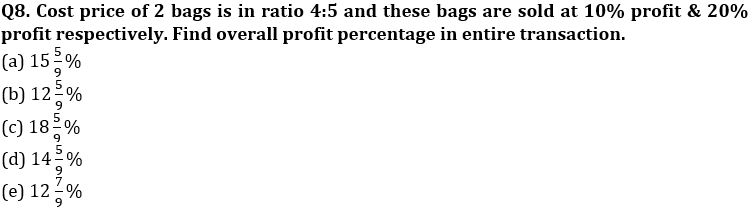Q9. Rs. 12000 becomes Rs. 15000 in 18 months at a certain rate of interest at simple interest. Find amount if Rs. 5000 invested at same rate for 30 months at simple interest.
(a) Rs. 7883.33
(b) Rs. 7083.33
(c) Rs. 7279.80
(d) Rs. 7173.33
(e) None of these

Q10.The interest earned on an amount after 2 yrs at 10 % per annum compounded yearly is Rs 672. Find the interest earned on same amount after 4 yr at 14 % per annum at simple interest ?
(a) Rs 1792
(b) Rs 1864
(c) Rs 1912
(d) Rs 1754
(e) Rs 1720

Q11.If the shopkeeper marked the price of an item 60% above the cost price and then gives two successive discount of 10% and 15% respectively, then find the profit percentage of the shopkeeper on selling the item?
(a) 25.4%
(b) 22.4%
(c) 20%
(d) 28.5%
(e) 32%

Q12. Neha invested an amount of Rs.6400 at the rate of R% p.a. on compound interest. If she gets a total amount of Rs. 1700 as interest at the end of 2 years, then find R%?
(a) 12.50%
(b) 25%
(c) 20%
(d) 17.50%
(e) 10%

Q13. P and Q start a business jointly. P invests Rs. 21000 for 9 months and Q invest Rs. X for 3 months. If Q gets two-third of total profit, then find the amount invested by Q. (in Rupees.)
(a) 63000
(b) 84000
(c) 42000
(d) 123000
(e) 126000

Q14. Mohan and Ravi started a business by investing Rs75000 and Rs45,000 respectively. After 8 Months Keshav Joined by investing Rs. X. If at the end of the year profit earned by Keshav is equal to average of profits earned by Mohan and Ravi together then find the value of X?
(a) 180000 Rs.
(b) 190000 Rs.
(c) 170000 Rs.
(d) 160000 Rs.
(e) 150000 Rs.

Q15. Shivam, Adarsh and Himanshu entered into a partnership business by investing Rs.6,000, Rs. X & Rs. (X – 4,000) respectively. The ratio of the period of investment of Shivam, Adarsh and Himanshu is 5:3:6 respectively. If the profit-sharing ratio of Shivam, Adarsh & Himanshu is 10:9:10 respectively, then find X.
(a) 6,000
(b) 7,000
(c) 10,000
(d) 9,000
(e) 8,000

Solutions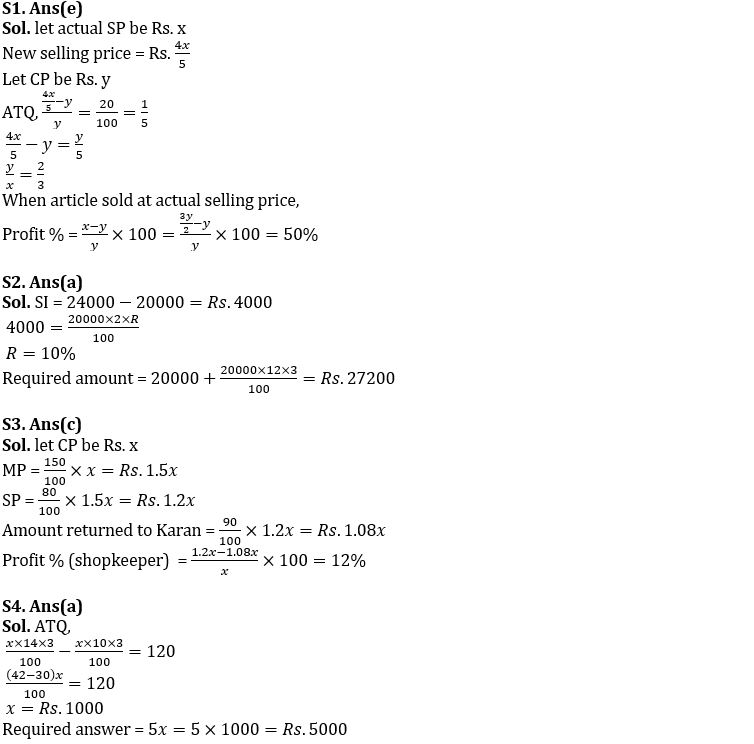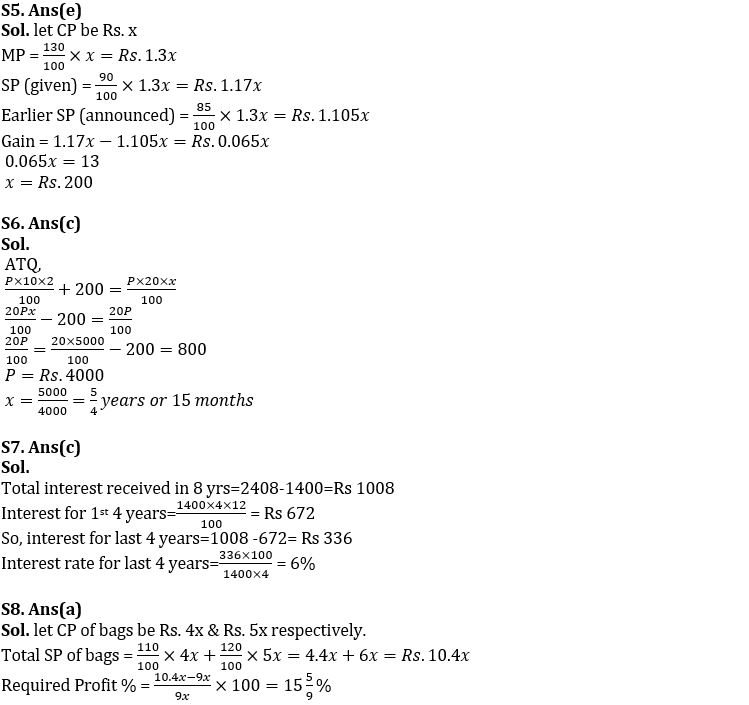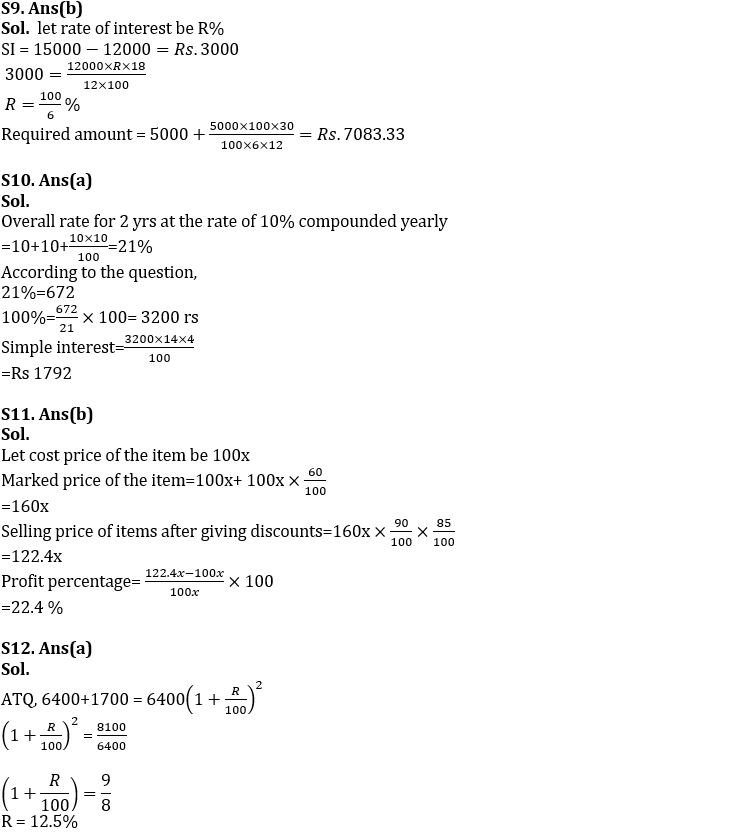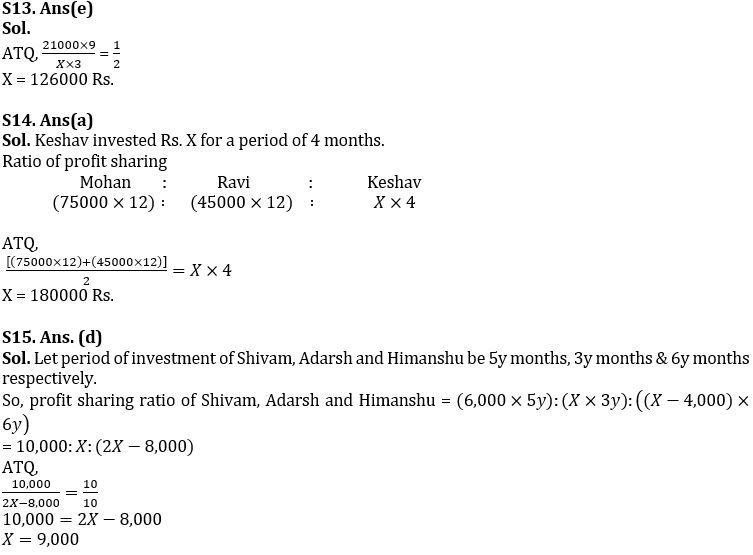#### Congratulations!Download Hindu Review of October 2021: Free PDF